# Quick start with R: Generalised Linear Model on binary data (Part 16)

In Part 15, we saw how to create a simple Generalised Linear Model on binary data using the `glm()` command. We continue with the same glm (modelling the vs variable on the weight and engine displacement) on the same data set – the `mtcars` dataset – which is built into R.
```model <- glm(formula = vs ~ wt + disp, data = mtcars, family = binomial) summary(model)```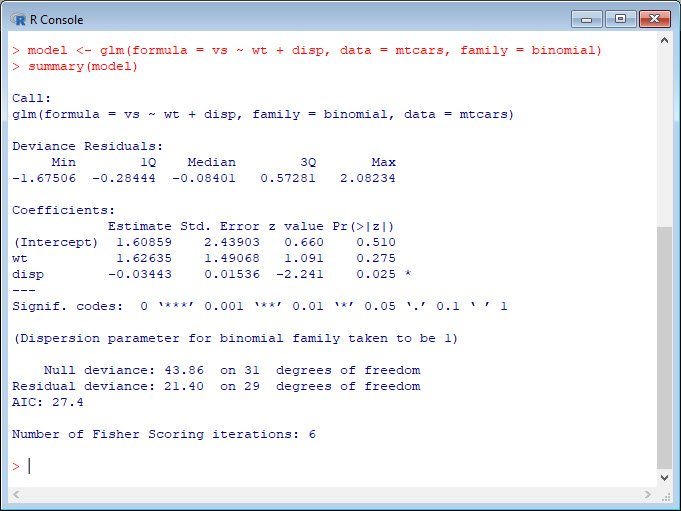We see that `weight` influences `vs` positively, while `displacement` has a slightly negative effect. We also see that the coefficient of `weight` is non-significant (p-value < 0.05) while the coefficient of `displacement` is significant. Later we will see how to investigate ways of improving our model.
However, the estimates (coefficients of the predictors `weight` and `displacement`) are now in units called logits. We will define the logit in a later blog.
We see the word Deviance twice over in the model output. Deviance is a measure of goodness of fit. R reports two forms of deviance – the null deviance and the residual deviance. The null deviance shows how well the response variable is predicted by a model that includes only the intercept (grand mean). For our example, we have a Chi square value of 43.9 on 31 degrees of freedom. This value indicates little fit (a significant difference between fitted values and observed values). Including the independent variables (`disp` and `weight`) decreased the deviance to 21.4 points on 29 degrees of freedom, so this Chi square value indicates a significant reduction in deviance. The Residual Deviance has reduced by 21.46 with a loss of three degrees of freedom. We have a very small (and therefore highly significant) p-value for the model.
`1 - pchisq(21.4, 3)`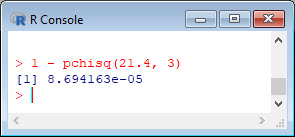Let us apply Hosmer and Lemeshow goodness of fit (GOF) test.
```library(ResourceSelection) hoslem.test(mtcars\$vs, fitted(model))```Unfortunately, we must reject the null hypothesis (i.e. our model) because the fitted values are significantly different from the data.
```plot(sort(model\$fitted), pch = 16, cex.lab = 1.3, ylim = c(0, 1)) points(sort(mtcars\$vs), pch = 19, col = "red", cex = 1.3)```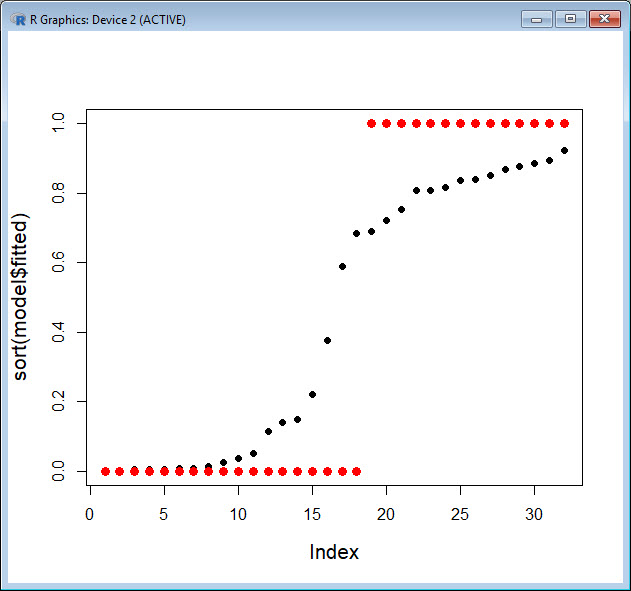Let’s try another plot, this time joining the fitted values with straight lines.
```plot(sort(mtcars\$vs), pch = 19, col = "red", cex = 1.3, ylab = "Proportion") points(sort(model\$fitted), ty = "o", pch = 16, cex.lab = 1.3, ylim = c(0, 1))```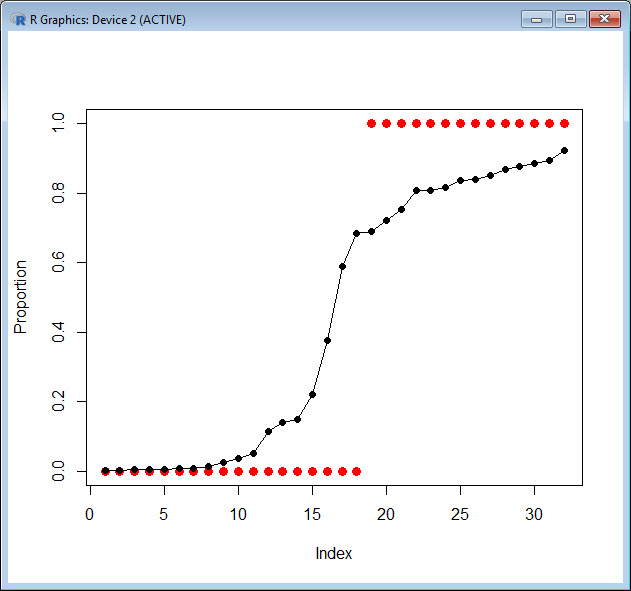The `glm()` has produced a kind of density that relates the observations for `vs = 0` to those for `vs = 1`.
We may wish to plot for each predictor separately, so first we fit a glm for `weight`.
```model_weight <- glm(formula = vs ~ wt, data = mtcars, family = binomial) summary(model_weight)````range(mtcars\$wt)````xweight <- seq(0, 6, 0.01) yweight <- predict(model_weight, list(wt = xweight),type="response") plot(mtcars\$wt, mtcars\$vs, pch = 16, xlab = "WEIGHT (g)", ylab = "VS") lines(xweight, yweight)```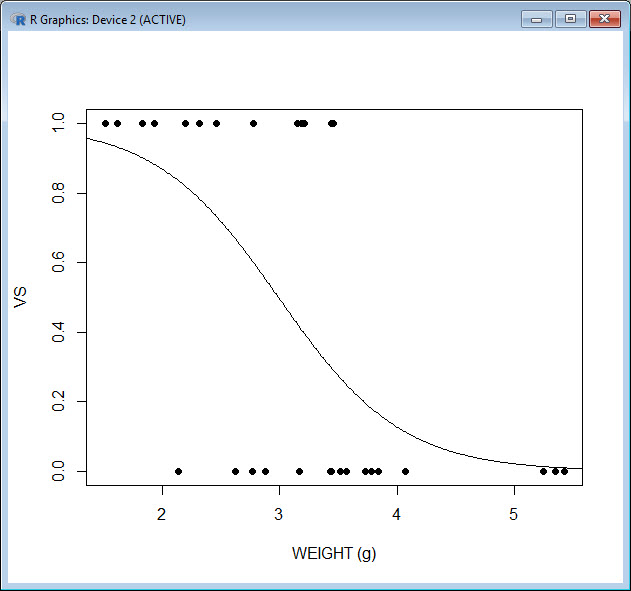```model_disp <- glm(formula = vs ~ disp, data = mtcars, family = binomial) summary(model_disp)```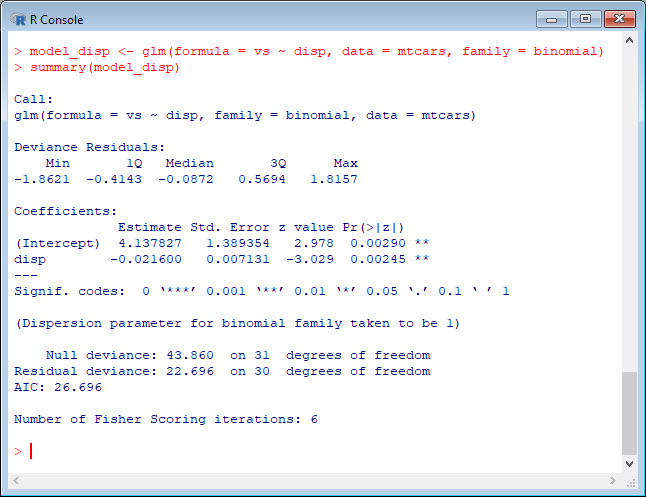`range(mtcars\$disp)`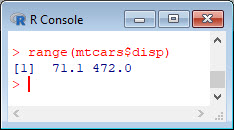```xdisp <- seq(0, 500, 0.01) ydisp <- predict(model_disp, list(disp = xdisp),type="response") plot(mtcars\$disp, mtcars\$vs, pch = 16, xlab = "DISPLACEMENT (cubic inches)", ylab = "VS") lines(xdisp, ydisp)```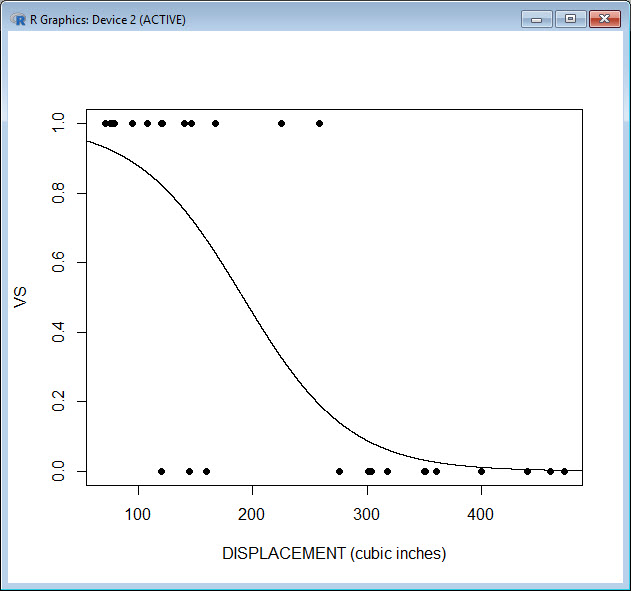That wasn’t so hard! In Blog 17 I will explain more about the output we got from the `glm()` function.
See you later!
David

#### Annex: R codes used

```# Estimate Generalised Linear Model and display results.
model <- glm(formula = vs ~ wt + disp, data = mtcars, family = binomial)
summary(model)

# Calculate Chi-square statistic.
1 - pchisq(21.4, 3)

library(ResourceSelection)

# Apply Hosmer and Lemeshow goodness of fit (GOF) test.
hoslem.test(mtcars\$vs, fitted(model))

# Graph estimated model.
plot(sort(model\$fitted), pch = 16, cex.lab = 1.3, ylim = c(0, 1))
points(sort(mtcars\$vs), pch = 19, col = "red", cex = 1.3)

# Alternative graph of the estimated model.
plot(sort(mtcars\$vs), pch = 19, col = "red", cex = 1.3, ylab = "Proportion")
points(sort(model\$fitted), ty = "o", pch = 16, cex.lab = 1.3, ylim = c(0, 1))

# Estimate Generalised Linear Model and display results.
model_weight <- glm(formula = vs ~ wt, data = mtcars, family = binomial)
summary(model_weight)

# Calculate range of values.
range(mtcars\$wt)

# Graph estimated model.
xweight <- seq(0, 6, 0.01)
yweight <- predict(model_weight, list(wt = xweight), type = "response")
plot(mtcars\$wt, mtcars\$vs, pch = 16, xlab = "WEIGHT (g)", ylab = "VS")
lines(xweight, yweight)

# Estimate Generalised Linear Model and display results.
model_disp <- glm(formula = vs ~ disp, data = mtcars, family = binomial)
summary(model_disp)

# Graph estimated model.
xdisp <- seq(0, 500, 0.01)
ydisp <- predict(model_disp, list(disp = xdisp), type = "response")
plot(mtcars\$disp, mtcars\$vs, pch = 16, xlab = "DISPLACEMENT (cubic inches)", ylab = "VS")
lines(xdisp, ydisp)
```